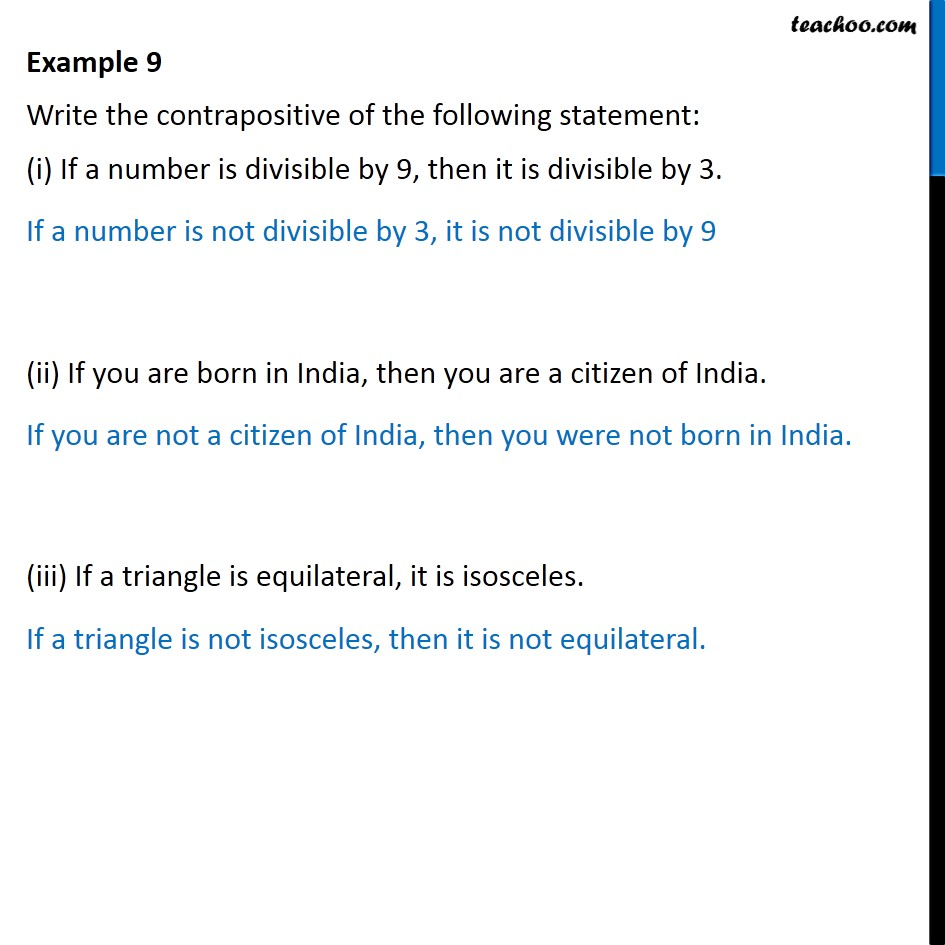1. Chapter 14 Class 11 Mathematical Reasoning (Deleted)
2. Serial order wise
3. Examples

Transcript

Example 9 Write the contrapositive of the following statement: (i) If a number is divisible by 9, then it is divisible by 3. If a number is not divisible by 3, it is not divisible by 9 (ii) If you are born in India, then you are a citizen of India. If you are not a citizen of India, then you were not born in India. (iii) If a triangle is equilateral, it is isosceles. If a triangle is not isosceles, then it is not equilateral.